Skip to main content

Advances, Systems and Applications

# Ground radar precipitation estimation with deep learning approaches in meteorological private cloud

## Abstract

Accurate precipitation estimation is significant since it matters to everyone on social and economic activities and is of great importance to monitor and forecast disasters. The traditional method utilizes an exponential relation between radar reflectivity factors and precipitation called Z-R relationship which has a low accuracy in precipitation estimation. With the rapid development of computing power in cloud computing, recent researches show that artificial intelligence is a promising approach, especially deep learning approaches in learning accurate patterns and appear well suited for the task of precipitation estimation, given an ample account of radar data. In this study, we introduce these approaches to the precipitation estimation, proposing two models based on the back propagation neural networks (BPNN) and convolutional neural networks (CNN) respectively, to compare with the traditional method in meteorological service systems. The results of the three approaches show that deep learning algorithms outperform the traditional method with 75.84% and 82.30% lower mean square errors respectively. Meanwhile, the proposed method with CNN achieves a better performance than that with BPNN for its ability to preserve the spatial information by maintaining the interconnection between pixels, which improves 26.75% compared to that with BPNN.

## Introduction

In recent years, the problem of climate change has caused the attention from all over the world. As one of the most significant factors in water resource ecosystem, precipitation plays an important role in meteorological fields, which has a strong impact on human’s daily lives as well as business such as agriculture and construction . The variations in time and quantity of rainfall have the potential impact on the agriculture yield and disaster management . Prior knowledge of rainfall behavior can help farmers and policy formulation to minimize crop damage. Moreover, it also plays an important role in disaster warning and relief .

The rain gauge is a simple and effective way to measure precipitation. However, the measurement network system is subject to many factors such as low density and the complexity of the precipitation phenomena would lead to a large error . With the advantage of the wide measurement range, high spatial and temporal resolution and the real-time data transmission, the ground radar has been widely applied in meteorological industry, including precipitation estimation . The traditional method employed in precipitation estimation is Z-R relationship model which utilizes the radar echo intensity and rainfall intensity to establish an equation to calculate the precipitation . The practical Z-R relationship is determined by the distribution of the droplet spectrum while the distribution is restricted by a lot of factors, which means that a constant Z-R relationship in a specific region would bring a large deviation on the precipitation estimation when applied in another region. Therefore, seeking for a more appropriate method is an inevitable approach to ensure the performance of the estimation .

Realizing the defect of the estimation method, many meteorologists have made great efforts to explore new methods. With the development of deep learning algorithms in recent years, studies using these methodologies have been drawing attention to improve the estimation performance [22, 23]. Deep learning is distinguished for the specialty in learning accurate relations from large and complex datasets, which is well suitable for the precipitation estimation task under elastic computing resources available in the cloud . Among the deep learning algorithms, the artificial neural network is a novel method simulating human’s thinking and memory based on the research of biological neural network. With the strong capacity of nonlinear mapping as well as its property of fault tolerance, adaptability and self-learning, the neural network becomes a new favorite to solve problems in the fields of precipitation estimation .

To address the issue in the accuracy of precipitation estimation mentioned above, back propagation neural networks and convolutional neural networks are applied to improve the accuracy of precipitation estimation. Especially, convolutional neural networks have not been applied in such research based on the data offered by the Doppler radar system. Then, there are extensive experimental evaluations to choose a more efficient and effective one of the proposed method. The specific objectives of this study are:

1) to introduce the deep learning methods based on the Doppler radar data to estimate precipitation in meteorological private cloud;

2) to compare the performances of the proposed methods with baseline model (Z-R relationship) and achieve a better method; and

3) to verify that whether the use of integrity radar data would enhance performance versus the discrete data.

The remainder of this paper is organized as follows: In “Related work” section, we review the peer research and work. “Data preparation” section describes the details of data preparation and the dataset employed in the experiment. The details of three models are presented in “Scheme” section. The experiments as well as their results and analysis are covered in “Experiments” section. Finally, we conclude our work in “Conclusion” section.

## Related work

With the great importance of the precipitation, many researchers have made efforts to estimate the precipitation as accurate as possible. In meteorology, Z-R relationship is a traditional method for estimation. The model reveals that radar reflectively factors have an exponential relation with precipitation, which is acquired from years of data. However, the accuracy of the model is of great deviation, especially unacceptable with a large error if the rain is heavy. In recent years, several approaches for precipitation estimation have been proposed in order to get a much better results.

Lazri et al.  developed a precipitation estimation scheme with the multi-layer perceptron (MLP) which utilized data from the high spectral resolution of the SEVIRI satellite. Two MLPs were used: MLP1 is used to identify rain and no-rain pixels and MLP2 is applied to estimate precipitation for rainfall pixels, which are beneficial for area-wide rainfall detection and quantification in a high spatial and temporal resolution.

Hernández et al.  introduced a deep learning architecture to estimate the accumulated precipitation for the next day. Their model includes an autoencoder and a multilayer perceptron. The autoencoder is an unsupervised network used to reduce and capture non-linear relationships between attributes and the multilayer perceptron is employed to make predictions in their problem. Compared with other previous proposals, it demonstrated that their model achieved an improvement on the prediction. However, the improvement is limited with single meteorological factors.

Francesco Beritelli et al.  proposed a new classification method applied to classify the precipitation into four rainfall intensities, which is based on a probabilistic neural network with three received signal level local features of the 4G/LTE. The performance of their model obtained an overall correct classification rate of 96.7%. However, their work was not further to estimate the specific precipitation.

Ouallouche et al.  introduced a precipitation estimation technique based on the random forest (RF) algorithm. The RF consists of two main parts: classification and regression, which are receptively performed on the MSG-retrieved data. The RF algorithm is applied to classify the MSG images to three classes, whereas the rainfall rate of the pixels is assigned to the convective and stratiform classes with the random forest regression. However, the night-time precipitation estimation was not as good as daytime precipitation scenes.

Pengcheng Zhang et al.  proposed a novel solution called Dynamic Regional Combined short-term rainfall Forecasting approach (DRCF) based on the Multi-layer Perceptron. They employed Principal Component Analysis to reduce the input dimension. After then, the output was put into a MLP to make the short-term rainfall forecasting. Moreover, they utilized the surrounding sites to predict the rainfall at the same time with the same process mentioned above. The final prediction was the average of the results from all sites. This method takes the high altitude weather information into consideration while the improvement of the performance is finite. Meanwhile, there are different amounts of sites in different areas, which make the accuracy of the prediction fluctuate greatly.

Folino et al.  proposed a universal model with machine learning technique based on a deep learning architecture, which integrates information derived from weather radars and satellites. The model consists of three components: Information Retrieval, Data Analytics and Evaluation and the model allows the combination of the information extracted by many data sources. The Evaluation component is based on a deep neural network to provide more accurate predictions for heavy rainfall cases with the weighted MAE loss function. The modified loss function narrowed the gap between the observation and the evaluation while the deep neural network is prone to overfit even the inverted dropout technique was employed.

Mojtaba et al.  proposed a CNN-based model with infrared and water vapor channels from geostationary satellites for precipitation estimation, which was compared with baseline models called PERSIANN-CCS and PERSIANN-SDAE through various evaluation indexes. Results demonstrated that the proposed model outperformed the baseline models as well as the efficiency. However, the estimation is the day precipitation that had no advantages in practical work because people pay more attention to the precipitation in a short time.

In order to enhance the improvement on precipitation estimation, we introduce two models based on the back propagation neural network and convolutional neural network respectively, which are compared with the traditional method of Z-R relationship, to find a better performance method.

## Data preparation

The data comes from a meteorological observatory located in the center area of Taizhou in Zhejiang province. The Doppler radar transmits radar reflectivity factors (dBZ) as well as the corresponding longitude and latitude every six minutes with eleven different elevation angles, which are stored with a binary file format orderly in private cloud . Meanwhile, there are four automatic weather stations recording the minutely precipitation ordered by date and time, which are considered as the authentic value to verify the accuracy of precipitation estimation. The data used in our experiments cover from 2013 to 2017.

The data used for models contain two main characteristics, including dBZ values and the corresponding precipitation from rain gauges. According to the meteorologists, the minimum elevation data are more closely associated with the precipitation. Therefore, the data from the minimum elevation were used in our experiments which were projected to a horizontal plane with the height of 1200 meters distant from the ground to achieve a high-precision and integral mosaic of dBZ, which is similarly regarded as a "square grid" with the resolution of 1km×1.

In order to utilize the radar reflectivity information for a better result, the area data instead of the single point value are taken into consideration. The center of the area matrix is the grid point nearest to the automatic weather station. Around the center, a 24km×24 area (with a total of 625 dBZ values on grid points) is employed as is shown in Fig. 1. In addition, due to the delay of the precipitation, the current value is substituted with the sum of next 6 minutes (including current precipitation) so that the precipitation would be more precise . As the unit of precipitation is mm/6min, it is necessary to transform the unit to mm/h. Therefore, each sample of data consists of two fields: a matrix with shape of 25×25 which memories the dBZ values as input and a one-hour precipitation as the authentic label of the model estimation.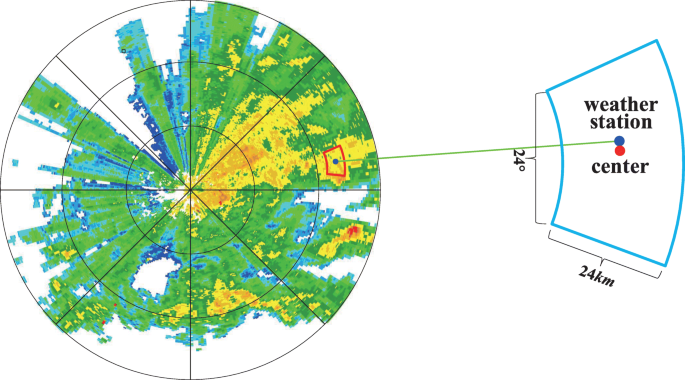Fig. 1

According to meteorological literatures above, the value of radar data (dBZ) below fifteen, which are also called ground echoes, hardly have an impact on the precipitation. Therefore, the average of the matrix elements below fifteen is to be abnegated which doesn’t contribute to the enhancement of the efficiency and accuracy.

The dataset is randomly divided into training set and test set with a percentage of 80 and 20 respectively, which means that the training set is employed to find the relationship between the radar reflectivity factors and precipitation so that the parameters are determined, while the test set is used to verify the accuracy of the relationship.

## Scheme

### Overview

When the artificial neural networks are applied to practical tasks, the main differences lie in the architecture and parameters of the networks. In order to find a better model to estimate precipitation, two models called Precipitation Estimation from Radar using Back Propagation Neural Network (PERBPNN) and Precipitation Estimation from Radar using Convolutional Neural Network (PERCNN) are proposed and conducted to compare the performance with the traditional methods of Z-R ralationship which is set as the baseline model. Figure 2 illustrates the overview of our scheme.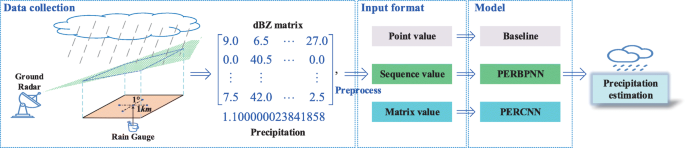Fig. 2

### Baseline model

For the moment, the precipitation with radar data in the industry is mainly calculated through the relationship between the radar echo intensity and rain intensity according to the Eq. (1):

$$Z = aR^{b}$$
(1)

where Z is the radar echo intensity, R is the one-hour precipitation and a, b are empirical coefficients. Due to the complexity of the meteorological problems, the coefficients may be diverse in different regions . Figure 3 shows the details of this method,especially there is a little different from original method that the average of the dBZ matrix is used instead of the single dBZ value in the center of the matrix. As a result, the effect of the surrounding is considered which would make a better performance.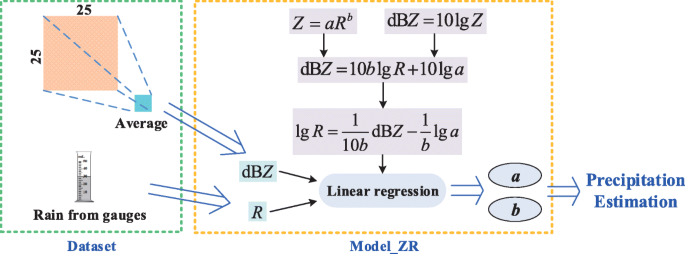Fig. 3

### PERBPNN

With the development of the hardware, deep learning becomes a new favorite which attracts scholars not only from the computer industry but from other industries, including meteorological industry. BPNN is one of the most representatives in deep learning . Once the data were put into the network, BPNN would optimize the parameters automatically. After some epochs of learning, the parameters would be determined automatically. Figure 4 shows the details of the BPNN. The dBZ matrix is reshaped to a column vector as the input of the model. Compared with the baseline model, concrete values in the dBZ matrix are employed in this model as it is the advantage of the BPNN which takes more features into consideration to enhance the estimation accuracy. The key component in the BPNN is the computation of each neuron which is expressed in Formula (2):

$$\left\{\begin{array}{l} \boldsymbol{z}^{[l]}=\boldsymbol{w}^{[l]}\boldsymbol{a}^{[l-1]}+\boldsymbol{b}^{[l]} \\ \boldsymbol{a}^{[l]}=g\left(\boldsymbol{z}^{[l]}\right) \end{array}\right. (l=1,2,\cdots,n)$$
(2)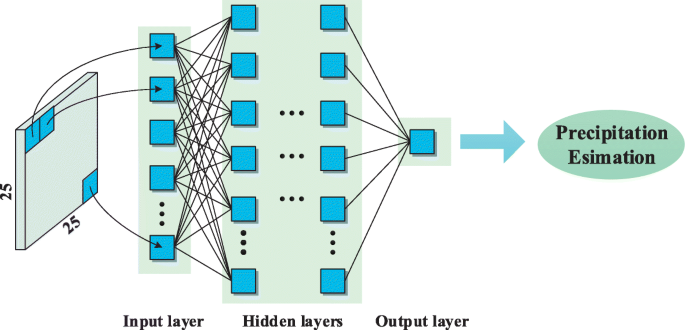Fig. 4

where n is the amount of layers, l represents that the variables belongs to the lth layer, w[l] is the parameters matrix and b[l] is the bias; a[l] is the output matrix of each layer, g is the suitable activation function. Therefore, the neurons of each layer are computed at the same time. The estimated value would be obtained through a series computation of hidden layers. Then, the stochastic gradient descent method which is shown in the Formula (3) is applied for the back propagation to adapt the parameters so that the accuracy becomes better [43, 44].

$$\left\{\begin{array}{l} \boldsymbol{w}^{[l]}=\boldsymbol{w}^{[l]}-\alpha\frac{\partial \boldsymbol{J}}{\partial \boldsymbol{w}^{[l]}} \\ \boldsymbol{b}^{[l]}=\boldsymbol{b}^{[l]}-\alpha\frac{\partial \boldsymbol{J}}{\partial \boldsymbol{b}^{[l]}} \end{array}\right. (l=1,2,\cdots,n)$$
(3)

where J is the loss function, α is the learning rate, w[l] and b[l] are the parameter matrix and bias vector in layer l. With some epochs of the forward propagation and back propagation, the final architecture of the model is determined.

### PERCNN

CNN is special for its ability to automatically extract features hierarchically. The use of convolutional kernel is capable of avoiding the one-to-one connections among all units and reducing the parameters with weight sharing. Moreover, it is beneficial to reduce the over-fitting as well as improve the computing speed and fault tolerance .

Figure 5 shows the details of PERCNN. The entirety of the dBZ matrix, which includes the neighborhood information, is applied as the input so that the features among the area could be extracted. When the dBZ matrix is put into the model, a lot of feature maps are calculated with several convolution and max-pooling operations. The output after each convolution is given in Formula (4):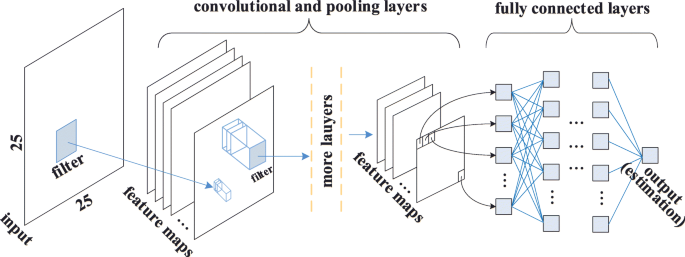Fig. 5
$$\left\{\begin{array}{l} \boldsymbol{z}_{i,j}^{[l]}=\sum\limits_{m=0}^{f-1}\sum\limits_{n=0}^{f-1}\boldsymbol{w}_{m,n}^{[l]}\boldsymbol{a}_{m+i,n+j}^{[l-1]}+\boldsymbol{b}^{[l]}\\ \boldsymbol{a}_{i,j}^{[l]}=g(\boldsymbol{z}_{i,j}^{[l]}) \end{array}\right.$$
(4)

where f is the kernel size, $$\boldsymbol {w}_{m,n}^{[l]}$$ is the weight at the position of (m,n) in the kernel, $$\boldsymbol {a}_{m+i,n+j}^{[l-1]}$$ is the value in the receptive field of layer l − 1 at the position of (m+i,n+j), and b[l] is the bias matrix of layer l, $$\boldsymbol {z}_{i,j}^{[l]}$$ is the direct result of each step of convolution in layer l, g is the suitable activation function and $$\boldsymbol {a}_{i,j}^{[l]}$$ is the ultimate output of layer l. Then, the size of the output is determined by Formula (5) as following:

$$n^{[l]}=\left\lfloor\frac{n^{[l-1]}+2p-f}{s}\right\rfloor+1$$
(5)

where n[l−1] and n[l] represent the size of feature from layer l − 1 and l respectively, f is the kernel size applied with stride s and padding p.

In addition, the pooling operation especially max pooling is usually employed to improve the robustness of feature extraction and reduce the dimension of the model between the convolution and activation function which is given in Formula (6):

$$\left\{\begin{array}{l} \boldsymbol{m}_{i,j}^{[l]}=\underset{(a,b)\in R_{p}}{max}\left(\boldsymbol{z}_{a,b}^{[l]}\right)\\ \boldsymbol{a}_{i,j}^{[l]}=g\left(\boldsymbol{m}_{i,j}^{[l]}\right) \end{array}\right.$$
(6)

where Rp represents the pooling domain of each stride, (a,b) represents the position in the pooling domain, $$\boldsymbol {m}_{i,j}^{[l]}$$ is the result of the max pooling and the remaining parameters are the same as the Formula (4).

Then, the ultimate feature maps of the first portion are squeezed to a one-dimensional vector to join the fully connected (FC) layers to estimate the precipitation. The FC networks are similar to the BPNN and the computation of the process is suitable to refer the Formula (2). And the output of the FC networks is the precipitation estimation.

After the forward propagation, the stochastic gradient descent algorithm is also employed to minimum the value of the loss function to achieve a better architecture, which is the same as the Formula (3). Experienced with some epochs of training, the final architecture of the model is determined.

## Experiments

With the private cloud built by the meteorological department as the platform, the experimental environment of this study was built. Data processing, model training and verification were completed in the private cloud.

Our networks were trained and tested on AMD Ryzen 5 3600 6-core Processor CPU and NVIDIA GeForce GTX 1660 6GB GPU. It took around 3 hours for 10000 epochs of training. During the experiments, we implemented a mini-batch system in order to adapt the restriction of the video memory. Each epoch of training consists of running all mini-batches to cover the training dataset. Meanwhile, some algrithms were applied to optimize the training process. We trained out model with PyTorch 1.3.1 framework(in python 3.6) which supports CUDA 10.0.

During the experiments, the mean square error (MSE) and root mean square error (RMSE) are applied as the loss function to estimate the performance of these models, which is shown in Formula (7):

\begin{aligned} &MSE=\frac{1}{m}\sum_{i=1}^{m}|\hat{y_{i}}-y_{i}|^{2} \\ &RMSE=\sqrt{MSE} \end{aligned}
(7)

where m is the sample size, $$\hat {y_{i}}$$ and yi respectively represent the estimated and authentic value of the sample i. The following is the specific experimental process.

### Baseline model

In this model, the dBZ matrix is transformed to a single value which is the average of 625 elements in the matrix. The relationship between dBZ and Z is shown in Eq. (8):

$$dBZ=10\lg Z$$
(8)

Then, the Formula (1) needs to be transformed to Eq. (9) as is shown below:

$$\lg Z=b\lg R+\lg a$$
(9)

More specifically, the Eq. (10) would be used for the linear regression:

$$\lg R=\frac{1}{10b}dBZ-\frac{1}{b}\lg a$$
(10)

Therefore, the least square method is used to solve the problem and the parameters as well as estimations are determined. The a is 0.762 and b is 0.003 through the computation.

### Neural network optimization

In order to make the performances of neural networks better and accelerate the training speed, several means are employed during the training.

#### Z-Score normalization

Z-Score normalization is a regularization method which transforms the input data into the standardized normal distribution as is shown in Formula (11). Standardization is essentially a linear transformation with many good properties, which determines that the change of data will not cause "failure", but improve the performance of data. It is beneficial to eliminate the effects caused by the differences of the value range, which makes the training speed faster and the estimation more accurate. This operation is conducted before the radar data are put into the model.

$$\left\{\begin{array}{l} \mu=\frac{1}{n}\sum\limits_{i=1}^{n}x_{i}\\ \sigma=\sqrt{\frac{1}{n}\sum\limits_{i=1}^{n}(x_{i}-\mu)^{2}}\\ x_{i}^{*}=\frac{x_{i}-\mu}{\sigma},i=1,2,\cdots,n \end{array}\right.$$
(11)

where n is the sample size, xi is the ith input, μ is the sample average, σ is the sample standard deviation and $$x_{i}^{*}$$ represents the normalization result of xi.

#### Batch normalization

This operation is similar to the Z-Score normalization. The difference is that the normalization is not applied on all data. It is used on the feature map before the convolution operation of each layer. Through the batch normalization, the problem of gradient disappearance is avoided, which means that the training speed becomes faster greatly. It is suitable for the batch normalization to apply Formula (11) as well, except that the n represents the count of the elements in the feature map.

#### Inverted dropout

During the training, it is easy to overfit, which makes the error of training set very low while the performance of test set is still poor. Inverted Dropout is a regularization technique that is able to reduce the overfitting of the training set effectively. It is applied on a hidden layer to set some weight as zero according to a certain probability, which is similar to delete some neuron nodes of the layer randomly during the forward propagation while the back propagation is not influenced. That is to say, the network is different during the forward propagation but the gradient descent works on the original network. This makes the parameters would not rely on the training set too much to reduce overfitting. The dropout rate is empirically set as 0.5.

### PERBPNN

The input layer and output layer are fixed with the number of neurons of 625 and 1 respectively. However, the structure of hidden layers is determined through trial and error. Table 1 presents the adopted parameters for the model with lots of tests.

Figure 6a displays the training and testing process. The orange curve and blue curve represent the relationship between loss and epoch on training set and test set respectively. It is shown that the training set loss decreased rapidly at the beginning and then fluctuated strongly when epoch increases. In addition, it is obvious that the test set loss becomes smooth and steady after 100 epochs. With lots of trials and tests, it is obvious that the number of epoch is suitable for 10000 since the loss would become relatively stable after 10000 epochs.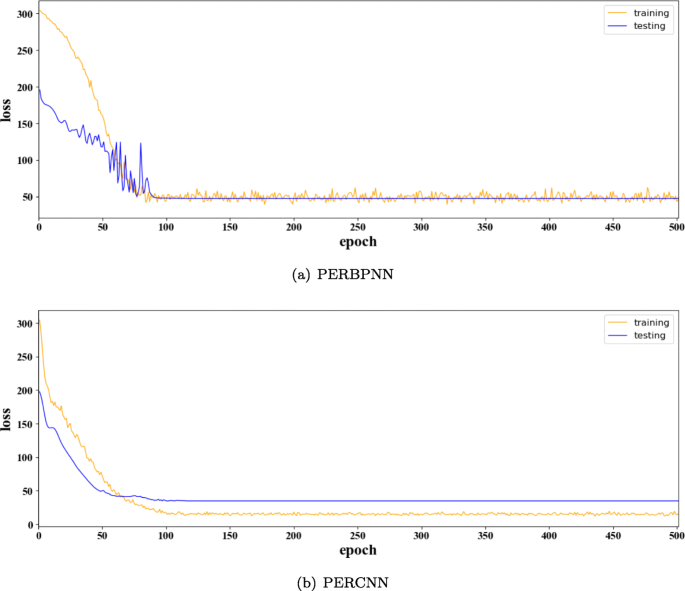Fig. 6

### PERCNN

The structure of PERCNN is determined by extensive trials as well. More specifically, a greedy strategy of search is conducted for the following set of parameters. Table 2 presents the approved values for each parameter.

It is noticeable that the pooling technique is not applied in our model because the pooling layer is not effective for estimation. The input of the model is a value matrix instead of an image matrix, so there are no textural features necessary to extract. For instance, we consider the three matrices with values representing the dBZ at some locations which is shown in the following:

$$\left [\begin{array}{lll} 1 & 1 & 1 \\ 1 & 5 & 1 \\ 1 & 1 & 1 \end{array} \right ], \left [\begin{array}{lll} 5 & 5 & 5 \\ 5 & 5 & 5 \\ 5 & 5 & 5 \end{array} \right ], \left [\begin{array}{lll} 4 & 6 & 4 \\ 2 & 8 & 2 \\ 4 & 6 & 4 \end{array} \right ]$$

Obviously, the three matrices are different from each other. However, if we apply a 2×2 max pooling on the matrices, the feature maps of the first two matrices becomes indistinguishable as follows:

$$\left [ \begin{array}{ll} 5 & 5 \\ 5 & 5 \end{array} \right ], \left [ \begin{array}{ll} 5 & 5 \\ 5 & 5 \end{array} \right ], \left [ \begin{array}{ll} 8 & 8 \\ 8 & 8 \end{array} \right ]$$

On the other side, if we apply a 2×2 average pooling on the matrices, the feature maps of the last two matrices becomes indistinguishable as well:

$$\left [ \begin{array}{ll} 2 & 2 \\ 2 & 2 \end{array} \right ], \left [ \begin{array}{ll} 5 & 5 \\ 5 & 5 \end{array} \right ], \left [ \begin{array}{ll} 5 & 5 \\ 5 & 5 \end{array} \right ]$$

And during the training, the performance of model without pooling technique is better than one with it. Therefore, the pooling technique is removed from our model.

The overview of the network is shown in Fig. 7. The specific configuration and parameter information of the whole network are detailed in Table 3. In order to make the result of the comparison with the PERBPNN, the activation function, optimizer and training times are the same as PERBPNN. The training and testing process is shown in Fig. 6b. Different from PERBPNN, after the rapid decrease at the beginning, both training set and test set losses are smooth and steady.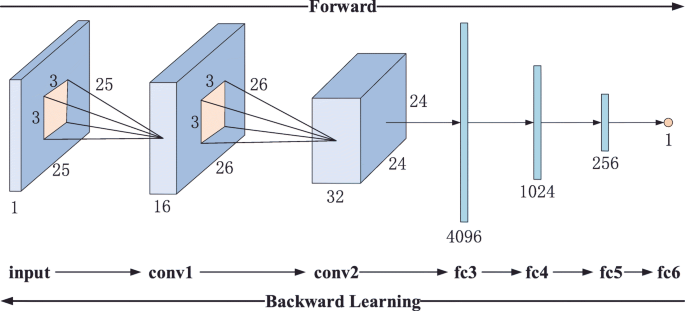Fig. 7

### Results

With the experiments among the three models, the research demonstrated that the estimation with the deep learning technique is really effective and it achieved a more accurate result as is presented in Fig. 8. It is clear that precipitation estimation from ground radar information acquired by PERBPNN and PERCNN correspond to the authentic value very well. The errors of each model are shown in Table 4 which accords with the results above.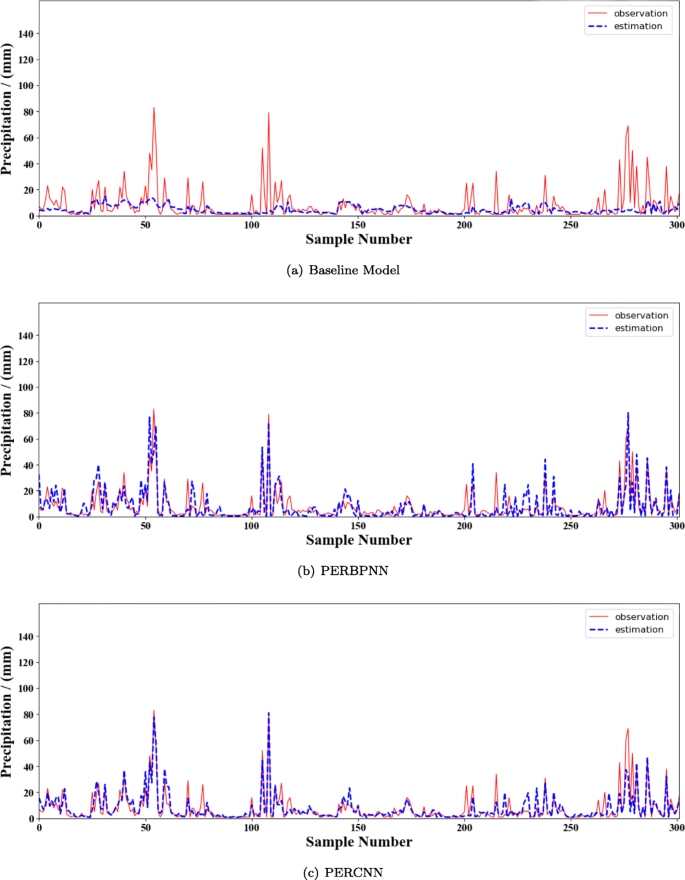Fig. 8

More specifically, the estimations are compared with the authentic values for those data instances. Figure 9 shows that the relation between estimation by the three models and the authentic precipitation. The x-axis represents the authentic value, while the y-axis represents the estimation. A good estimation would be place in a straight line with the values of slope equal and intercept equal to one and zero respectively. As it is possible to observe, the distribution of the data points from deep learning models are located around the aforementioned reference line while the traditional method have a poor performance. Obviously, the performance with CNN is the best, the second is BPNN and the Z-R model is a little unsatisfactory. The baseline model performed well just when the precipitation is in a low level while it presented with a big bias as the precipitation increases. Instead, PERBPNN and PERCNN almost have a good performance almost all the time, and the latter is more accurate as the data points of PERCNN are closer to the reference line which is illustrated in the graph. More specifically, the neighbor data of the center is of great effect on the estimation accuracy. It is observed that BPNN captures the contribution of single data to the estimation while leaving out the integrity of the data. However, precipitation is a continuous process since there is no possibility that only a small area rains heavily while the neighbor area is suddenly no rain. Therefore, CNN is more suitable for the estimation due to the ability of integrity feature extraction.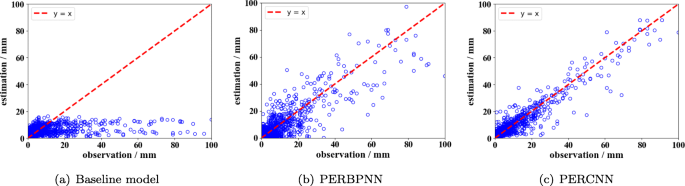Fig. 9

## Conclusion

In this study, we implemented three different models to estimate the precipitation from ground radar information. Experimental results showed that the performance of deep learning models are better than that of the traditional models. In addition, the RMSE of PERCNN is reduced by 14.41% compared with PERBPNN. It indicated that precipitation estimation with surrounding radar data achieved a more accurate result especially the integration feature of neighborhood information. In the future, we will explore more effective methods to enhance the accuracy of the estimation and try to study the precipitation prediction.

## Availability of data and materials

The raw data required to reproduce these findings cannot be shared at this time as the data also forms part of an ongoing study.

## References

1. 1

Kong C, Luo G, Tian L, Cao X (2018) Disseminating authorized content via data analysis in opportunistic social networks. Big Data Min Anal 2(1):12–24.

2. 2

Kumar S, Singh M (2018) Big data analytics for healthcare industry: impact, applications, and tools. Big Data Min Anal 2(1):48–57.

3. 3

He JS, Han M, Ji S, Du T, Li Z (2019) Spreading social influence with both positive and negative opinions in online networks. Big Data Min Anal 2(2):100–117.

4. 4

McMichael AJ, Woodruff RE, Hales S, et al. (2006) Climate change and human health: present and future risks. The Lancet 367(9513):859–869. Elsevier.

5. 5

Tian W, Ma T, Zheng Y, Wang X, Tian Y, Al-Dhelaan A, Al-Rodhaan M (2015) Weighted curvature-preserving pde image filtering method. Comput Math Appl 70(6):1336–1344.

6. 6

Zhang Y, Ge T, Tian W, Liou Y-A (2019) Debris flow susceptibility mapping using machine-learning techniques in shigatse area, china. Remote Sens 11(23):2801.

7. 7

Trenberth KE, Dai A, Rasmussen RM, Parsons DB (2003) The changing character of precipitation. Bull Am Meteorol Soc 84(9):1205–1218.

8. 8

Zhou J, Wang T, Cong P, Lu P, Wei T, Chen M (2019) Cost and makespan-aware workflow scheduling in hybrid clouds. J Syst Archit 100:101631.

9. 9

Qi L, He Q, Chen F, Dou W, Wan S, Zhang X, Xu X (2019) Finding all you need: Web apis recommendation in web of things through keywords search. IEEE Trans Comput Soc Syst. https://doi.org/10.1109/tcss.2019.2906925.

10. 10

Gehne M, Hamill TM, Kiladis GN, Trenberth KE (2016) Comparison of global precipitation estimates across a range of temporal and spatial scales. J Climate 29(21):7773–7795.

11. 11

Huffman GJ, Adler RF, Morrissey MM, Bolvin DT, Curtis S, Joyce R, McGavock B, Susskind J (2001) Global precipitation at one-degree daily resolution from multisatellite observations. J Hydrometeorol 2(1):36–50.

12. 12

Zhou J, Hu XS, Ma Y, Sun J, Wei T, Hu S (2019) Improving availability of multicore real-time systems suffering both permanent and transient faults. IEEE Trans Comput 68(12):1785–1801.

13. 13

Bouazizi M, Ohtsuki T (2019) Multi-class sentiment analysis on twitter: Classification performance and challenges. Big Data Min Anal 2(3):181–194.

14. 14

Wang S, Zhou A, Bao R, Chou W, Yau SS (2018) Towards green service composition approach in the cloud. IEEE Trans Serv Comput. https://doi.org/10.1109/tsc.2018.2868356.

15. 15

Xu X, Li Y, Huang T, Xue Y, Peng K, Qi L, Dou W (2019) An energy-aware computation offloading method for smart edge computing in wireless metropolitan area networks. J Netw Comput Appl 133:75–85.

16. 16

Campos E, Zawadzki I (2000) Instrumental uncertainties in z–r relations. J Appl Meteorol 39(7):1088–1102.

17. 17

Gong W, Qi L, Xu Y (2018) Privacy-aware multidimensional mobile service quality prediction and recommendation in distributed fog environment. Wirel Commun Mobile Comput 2018. https://doi.org/10.1155/2018/3075849.

18. 18

Xu X, Xue Y, Qi L, Yuan Y, Zhang X, Umer T, Wan S (2019) An edge computing-enabled computation offloading method with privacy preservation for internet of connected vehicles. Futur Gener Comput Syst 96:89–100.

19. 19

Arnaud P, Bouvier C, Cisneros L, Dominguez R (2002) Influence of rainfall spatial variability on flood prediction. J Hydrol 260(1-4):216–230.

20. 20

Xu X, Fu S, Qi L, Zhang X, Liu Q, He Q, Li S (2018) An iot-oriented data placement method with privacy preservation in cloud environment. J Netw Comput Appl 124:148–157.

21. 21

Liu H, Kou H, Yan C, Qi L (2019) Link prediction in paper citation network to construct paper correlation graph. EURASIP J Wirel Commun Netw 2019(1):1–12.

22. 22

Wang S, Zhou A, Yang M, Sun L, Hsu C-H, et al. (2017) Service composition in cyber-physical-social systems. IEEE Trans Emerg Top Comput.

23. 23

Zhou J, Sun J, Cong P, Liu Z, Wei T, Zhou X, Hu SSecurity-Critical Energy-Aware Task Scheduling for Heterogeneous Real-Time MPSoCs in IoT. in press. https://doi.org/10.1109/tsc.2019.2963301.

24. 24

Liu W, Wang Z, Liu X, Zeng N, Liu Y, Alsaadi FE (2017) A survey of deep neural network architectures and their applications. Neurocomputing 234:11–26.

25. 25

Xu X, Liu Q, Luo Y, Peng K, Zhang X, Meng S, Qi L (2019) A computation offloading method over big data for iot-enabled cloud-edge computing. Futur Gener Comput Syst 95:522–533.

26. 26

Qi L, Zhang X, Dou W, Hu C, Yang C, Chen J (2018) A two-stage locality-sensitive hashing based approach for privacy-preserving mobile service recommendation in cross-platform edge environment. Futur Gener Comput Syst 88:636–643.

27. 27

Litjens G, Kooi T, Bejnordi BE, Setio AAA, Ciompi F, Ghafoorian M, Van Der Laak JA, Van Ginneken B, Sánchez CI (2017) A survey on deep learning in medical image analysis. Med Image Anal 42:60–88.

28. 28

Darji MP, Dabhi VK, Prajapati HB (2015) Rainfall forecasting using neural network: A survey In: 2015 International Conference on Advances in Computer Engineering and Applications, 706–713.. IEEE. https://doi.org/10.1109/icacea.2015.7164782.

29. 29

Nayak DR, Mahapatra A, Mishra P (2013) A survey on rainfall prediction using artificial neural network. Int J Comput Appl 72(16).

30. 30

Lazri M, Ameur S, Mohia Y (2014) Instantaneous rainfall estimation using neural network from multispectral observations of seviri radiometer and its application in estimation of daily and monthly rainfall. Adv Space Res 53(1):138–155.

31. 31

Hernández E, Sanchez-Anguix V, Julian V, Palanca J, Duque N (2016) Rainfall prediction: A deep learning approach In: International Conference on Hybrid Artificial Intelligence Systems, 151–162.. Springer. https://doi.org/10.1007/978-3-319-32034-2_13.

32. 32

Beritelli F, Capizzi G, Sciuto GL, Napoli C, Scaglione F (2018) Rainfall estimation based on the intensity of the received signal in a lte/4g mobile terminal by using a probabilistic neural network. IEEE Access 6:30865–30873.

33. 33

Ouallouche F, Lazri M, Ameur S (2018) Improvement of rainfall estimation from msg data using random forests classification and regression. Atmos Res 211:62–72.

34. 34

Zhang P, Jia Y, Gao J, Song W, Leung HK (2018) Short-term rainfall forecasting using multi-layer perceptron. IEEE Trans Big Data. https://doi.org/10.1109/tbdata.2018.2871151.

35. 35

Folino G, Guarascio M, Chiaravalloti F, Gabriele S (2019) A deep learning based architecture for rainfall estimation integrating heterogeneous data sources In: 2019 International Joint Conference on Neural Networks (IJCNN), 1–8.. IEEE. https://doi.org/10.1109/ijcnn.2019.8852229.

36. 36

Sadeghi M, Asanjan AA, Faridzad M, Nguyen P, Hsu K, Sorooshian S, Braithwaite D (2019) Persiann-cnn: Precipitation estimation from remotely sensed information using artificial neural networks–convolutional neural networks. J Hydrometeorol 20(12):2273–2289.

37. 37

Xu X, Mo R, Dai F, Lin W, Wan S, Dou W (2019) Dynamic resource provisioning with fault tolerance for data-intensive meteorological workflows in cloud. IEEE Trans Ind Inform. https://doi.org/10.1109/tii.2019.2959258.

38. 38

Qi L, Chen Y, Yuan Y, Fu S, Zhang X, Xu X (2019) A qos-aware virtual machine scheduling method for energy conservation in cloud-based cyber-physical systems. World Wide Web:1–23. https://doi.org/10.1007/s11280-019-00684-y.

39. 39

Wang S, Zhao Y, Xu J, Yuan J, Hsu C-H (2019) Edge server placement in mobile edge computing. J Parallel Distrib Comput 127:160–168.

40. 40

Gosset M, Kunstmann H, Zougmore F, Cazenave F, Leijnse H, Uijlenhoet R, Chwala C, Keis F, Doumounia A, Boubacar B, et al. (2016) Improving rainfall measurement in gauge poor regions thanks to mobile telecommunication networks. Bull Am Meteorol Soc 97(3):49–51.

41. 41

Wu W, Zou H, Shan J, Wu S (2018) A dynamical zr relationship for precipitation estimation based on radar echo-top height classification. Adv Meteorol 2018. https://doi.org/10.1155/2018/8202031.

42. 42

Hameed AA, Karlik B, Salman MS (2016) Back-propagation algorithm with variable adaptive momentum. Knowl Based Syst 114:79–87.

43. 43

Bottou L (2012) Stochastic gradient descent tricks In: Neural Networks: Tricks of the Trade, 421–436.. Springer. https://doi.org/10.1007/978-3-642-35289-8_25.

44. 44

LeCun YA, Bottou L, Orr GB, Müller K-R (2012) Efficient backprop In: Neural Networks: Tricks of the Trade, 9–48.. Springer. https://doi.org/10.1007/3-540-49430-8_2.

45. 45

McCann MT, Jin KH, Unser M (2017) Convolutional neural networks for inverse problems in imaging: A review. IEEE Signal Process Mag 34(6):85–95.

46. 46

Zhang L, Suganthan PN (2016) A survey of randomized algorithms for training neural networks. Inf Sci 364:146–155.

47. 47

Li Y, Hao Z, Lei H (2016) Survey of convolutional neural network. J Comput Appl 36(9):2508–2515.

Download references

## Author information

Authors

### Contributions

The authors equally contributed to this research and the paper initiated by the corresponding author. All authors read and approved the final manuscript.

### Corresponding author

Correspondence to Wei Tian.

## Ethics declarations

### Competing interests

The authors declare that they have no competing interests.

## Additional information

### Publisher’s Note

Springer Nature remains neutral with regard to jurisdictional claims in published maps and institutional affiliations.

## Rights and permissions

Open Access This article is licensed under a Creative Commons Attribution 4.0 International License, which permits use, sharing, adaptation, distribution and reproduction in any medium or format, as long as you give appropriate credit to the original author(s) and the source, provide a link to the Creative Commons licence, and indicate if changes were made. The images or other third party material in this article are included in the article’s Creative Commons licence, unless indicated otherwise in a credit line to the material. If material is not included in the article’s Creative Commons licence and your intended use is not permitted by statutory regulation or exceeds the permitted use, you will need to obtain permission directly from the copyright holder. To view a copy of this licence, visit http://creativecommons.org/licenses/by/4.0/.

Reprints and Permissions